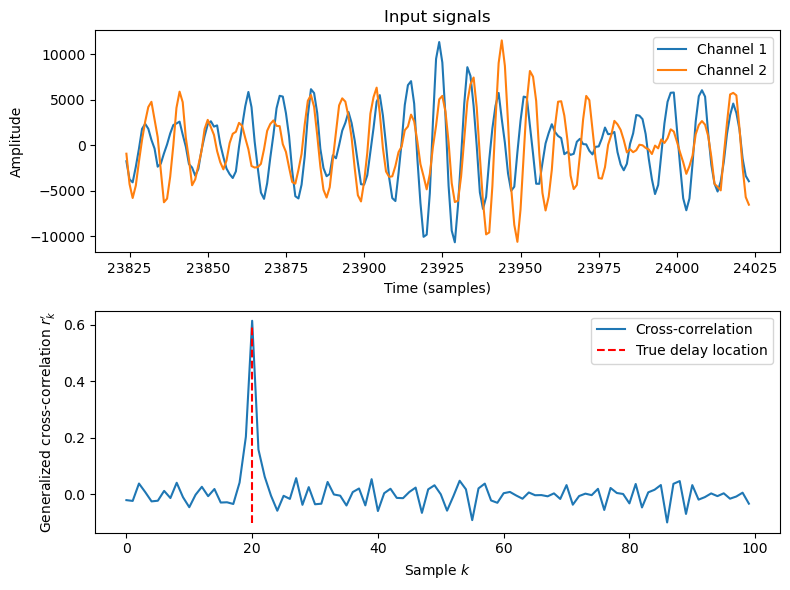# 11.8.3. Time-Delay of Arrival (TDoA) and Direction of Arrival (DoA) Estimation#

In multi-channel speech enhancement, a regularly appearing task is to estimate the time-delay between channels or equivalently, the angle at which a wavefront arrives to an array of microphones. By knowing the time-delay or angle of arrival, we can use beamforming to isolate sounds from that particular direction. A frequently used method for time-delay estimation is the generalized cross-correlation (GCC) method and especially its PHAT-weighted variant known as GCC-PHAT .

## 11.8.3.1. Generalized cross-correlation#

The cross-spectrum of two spectra $$X_{1,k,t}$$ and $$X_{2,k,t}$$ is

$C_{k,t} = X_{1,k,t}^* X_{2,k,t},$

where $$k$$ and $$t$$ are the frequency and time indices. The spectra are of form $$X_{h,k}=a_{h,k} e^{i\frac{2\pi kn_h}N}$$, where $$n_h$$ is the time-offset and $$N$$ is the length of the analysis window. We thus have

$C_k = a_{1,k} e^{-i\frac{2\pi kn_1}N} a_{2,k} e^{i\frac{2\pi kn_2}N} .$

If the time-difference between channels is $$\tau=n_2-n_1$$, then

$C_k = a_{1,k}a_{2,k} e^{-i\frac{2\pi kn_1}N+i\frac{2\pi k(\tau+n_1)}N} = a_{1,k}a_{2,k} e^{i\frac{2\pi k\tau}N} .$

It can be weighted with a variety of approaches such as

$C_k' = \frac{X_{1,k}^* X_{2,k}}{|X_{1,k}^* X_{2,k}|} = e^{i\frac{2\pi k\tau}N}$

to obtain the generalized cross-spectrum. The generalized cross-correlation is the inverse Fourier transform of the generalized cross-spectrum

$r_k' = {\mathcal F}^{-1}\{C_k'\} = \delta_\tau,$

where $$\delta_k$$ is the Dirac-delta function. In other words, the generalized cross-covariance has a single peak whose position indicates the time-delay $$\tau$$ between the two channels.

Hide code cell source
import numpy as np
import matplotlib.pyplot as plt
from scipy.io import wavfile
import scipy
import IPython.display as ipd
from helper_functions import stft

filename = 'sounds/temp.wav'

delay_samples = 20
delay_zeros = np.zeros(delay_samples)

data1 = np.concatenate((data,delay_zeros))
data2 = np.concatenate((delay_zeros,data))

noise_gain_dB = -10
noise_gain = np.std(data)*10**(noise_gain_dB/20)

observation1 = data1 + noise_gain*np.random.randn(len(data1))
observation2 = data2 + noise_gain*np.random.randn(len(data1))

X1 = stft(observation1,fs)
X2 = stft(observation2,fs)

crossspectrum = np.mean(np.conj(X1)*X2,axis=0)
crosscorrelation = scipy.fft.irfft(crossspectrum/np.abs(crossspectrum))

Hide code cell source
plt.figure(figsize=[8,6])
plt.subplot(211)
ix = np.argmax(observation1)
plt.plot(range(ix-100,ix+100),observation1[(ix-100):(ix+100)],label='Channel 1')
plt.plot(range(ix-100,ix+100),observation2[(ix-100):(ix+100)],label='Channel 2')
plt.xlabel('Time (samples)')
plt.ylabel('Amplitude')
plt.legend()
plt.title('Input signals')

plt.subplot(212)
plt.plot(crosscorrelation[0:100],label='Cross-correlation')
plt.plot([delay_samples,delay_samples],[-.1,.6],'r--',label='True delay location')
plt.legend()
plt.xlabel('Sample $k$')
plt.ylabel("Generalized cross-correlation $r_k'$")

plt.tight_layout()
plt.show()